Type a math problemalgebra
trigonometry
statistics
calculus
matrices
variables
list
AC
log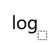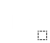ln
(
)
7
8
9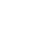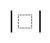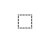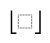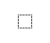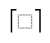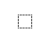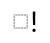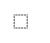τ
π
4
5
6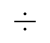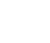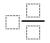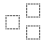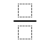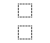%
θ
1
2
3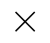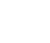<
>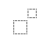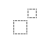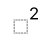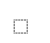x
i
0
.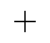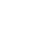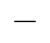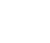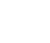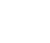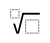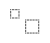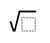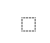y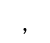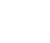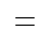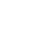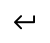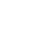EvaluateSteps For Long MultiplicationFirst line up the numbers vertically and match the places from the right like this.
Now multiply the first number with the digit in number to get intermediate results. That is Multiply 699 with 3. Write the result 2097 at the end leaving 0 spaces to the right like this.
Now multiply the first number with the digit in number to get intermediate results. That is Multiply 699 with 3. Write the result 2097 at the end leaving 1 spaces to the right like this.
Now multiply the first number with the digit in number to get intermediate results. That is Multiply 699 with 5. Write the result 3495 at the end leaving 2 spaces to the right like this.
View solution steps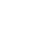## Similar Problems from Web Search

\begin{array}{c}\phantom{\times999}699\\\underline{\times\phantom{999}533}\\\end{array}
First line up the numbers vertically and match the places from the right like this.
\begin{array}{c}\phantom{\times999}699\\\underline{\times\phantom{999}533}\\\phantom{\times99}2097\\\end{array}
Now multiply the first number with the 1^{st} digit in 2^{nd} number to get intermediate results. That is Multiply 699 with 3. Write the result 2097 at the end leaving 0 spaces to the right like this.
\begin{array}{c}\phantom{\times999}699\\\underline{\times\phantom{999}533}\\\phantom{\times99}2097\\\phantom{\times9}2097\phantom{9}\\\end{array}
Now multiply the first number with the 2^{nd} digit in 2^{nd} number to get intermediate results. That is Multiply 699 with 3. Write the result 2097 at the end leaving 1 spaces to the right like this.
\begin{array}{c}\phantom{\times999}699\\\underline{\times\phantom{999}533}\\\phantom{\times99}2097\\\phantom{\times9}2097\phantom{9}\\\underline{\phantom{\times}3495\phantom{99}}\\\end{array}
Now multiply the first number with the 3^{rd} digit in 2^{nd} number to get intermediate results. That is Multiply 699 with 5. Write the result 3495 at the end leaving 2 spaces to the right like this.
\begin{array}{c}\phantom{\times999}699\\\underline{\times\phantom{999}533}\\\phantom{\times99}2097\\\phantom{\times9}2097\phantom{9}\\\underline{\phantom{\times}3495\phantom{99}}\\\phantom{\times}372567\end{array}